# P Pi D Pi Bonds for JEE## Overlapping of Orbitals

The process in which two atoms come so close to each other that they penetrate each other's orbital and produce a new hybridised orbital in which the bonding pair of electrons resides is referred to as overlapping of orbitals. Since the hybridised orbital has less energy than the atomic orbital, it is stable and is in its lowest energy condition. Orbital overlap is the term for this partial penetration of the orbital.

The amount of overlap is determined by the size and valence electrons of the two involved atoms. In general, the stronger the link created between the two atoms, the higher the overlap. As a result of the orbital overlap idea, atoms interact by overlapping their orbitals, generating a lower energy state in which their valence electrons with opposing spin team up to create a covalent bond.

## Sigma Bond

Head-on-head (same phase) overlap of atomic orbitals along the internuclear axis forms this sort of covalent bond. Due to the direct overlapping of the participating orbitals, sigma bonds are the strongest covalent bonds. The electrons that participate in a bond are referred to as sigma electrons.

A sigma bond is a bond produced by the end-to-end overlap of orbitals, with the electron density centred between the bonding atomic nuclei.

The methane molecule, for example, has four C-H sigma bonds. The overlap of bonding orbitals along the internuclear axis forms this type of covalent bond.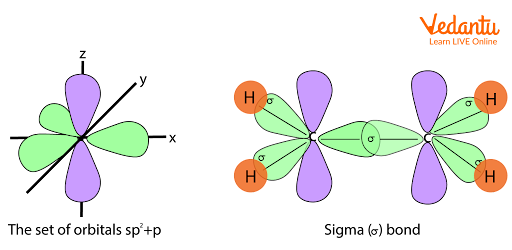Overlapping in sigma bond

## Pi Bond

Pi bonds are covalent chemical bonds that involve the lateral or sidewise overlapping of two lobes of one atomic orbital with two lobes of another atomic orbital from a different atom. The Greek letter " $\pi$ " relates to the comparable symmetry of the pi bond and the p orbital, which is why pi bonds are sometimes written as  ‘$\pi$ bonds'. Almost always, pi bonds are weaker than the sigma bonds.

A single sigma bond and a single pi bond make up a double bond in most cases.

An example of pi bonds can be seen in ethene.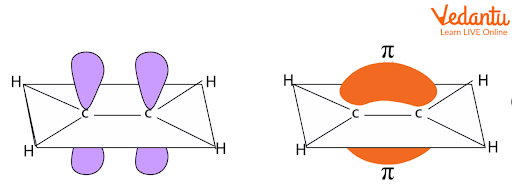Overlap of p orbitals leading to formation of pi  bond

P orbitals are commonly used in pi bonding. d orbitals, on the other hand, can engage in bonding and form multiple bonds using d orbitals between two atoms.

## What is P Pi P Pi Bond?

The p-orbitals of one atom overlap sideways with the p-orbitals of another atom in this type of bonding, resulting in the formation of a pi bond, also known as a p pi p pi bond. Examples include CO, CN

## P Pi D Pi Bond

If there is a bond between two atoms with one vacant orbital on one atom and one lone pair of electrons on the other atom, then this electron pair is donated to that corresponding unoccupied orbital, and the bonding is called P pi d pi bonding.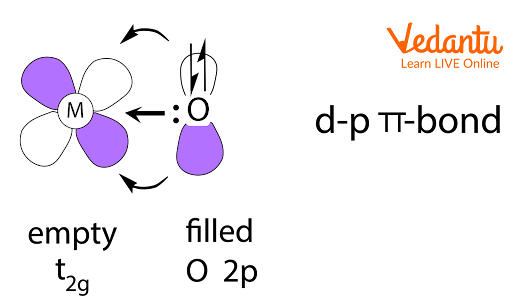p pi d pi bond example

The p pi d pi bond is stronger than the p pi p pi bond.

Due to a larger level of overlap in the electron clouds in the p pi d pi bond, the extent of overlap is greater, resulting in a lower energy bonding MO.

When the pi-bond is established between the p-orbital of one element and the d-orbital of the other element, it is called the d pi p pi bond. The d pi p pi bond cannot be formed by elements that do not have a vacant d-orbital. As a result, period 2 elements do not have unoccupied d-orbitals and only have vacant p-orbitals, allowing them to form multiple pi bonds. Period 3 and above elements, on the other hand, have an empty d-orbital and can create d pi p pi multiple bonds.

P pi d pi bonding examples:

## PO43−

This molecule is made up of two elements: Phosphorus atoms and Oxygen atoms.  Since the oxygen element belongs to period 2 and the phosphorus element to period 3, it can form p pi d pi multiple bonds.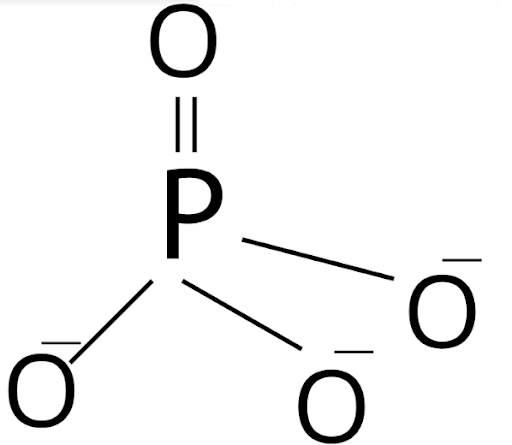d pi p pi bond in PO43−

## SO43-

This molecule is made up of two elements: Sulphur atoms and Oxygen atoms. Since the oxygen element belongs to period 2 and the sulphur element to period 3, it can form p pi d pi multiple bonds.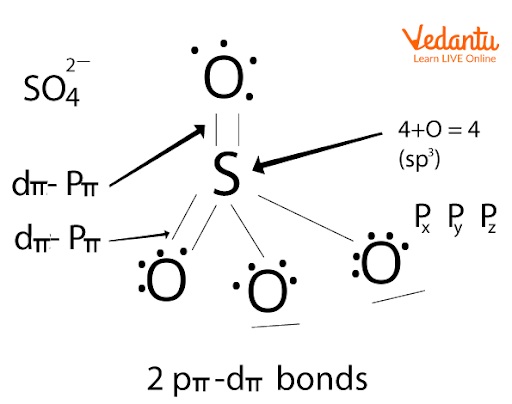p pi d pi bonds in SO43-

## Conclusion

The process in which two atoms come so close to each other that they penetrate each other's orbital and produce a new hybridised orbital is referred to as overlapping of orbitals. Pi bonds are covalent chemical bonds that involve the lateral overlapping of two lobes of one atomic orbital with two lobes of another atomic orbital from a different atom. If there is a bond between two atoms with one vacant orbital on one atom and one lone pair of electrons on the other atom, then this electron pair is donated to that corresponding unoccupied orbital, and the bonding is known as p(pi) - d. (pi) bonding. Eg PO43−

## FAQs on P Pi D Pi Bonds for JEE

1. Which molecule has only pi bonds?

According to molecular orbital theory, C2 is a diatomic molecular species with just pi bonds.

The bond order on C2 is two due to the presence of four electrons in its pi bonding orbital. Also, the pi bonds here are stronger than sigma bonds due to the lower energy of bonding pi molecular orbitals, as shown in the molecular orbital diagram of C2 , which contains a pi bond.

In a degenerate pi bonding set of orbitals, MO Theory reveals that there are two sets of paired electrons. The paired electrons are in the pi orbitals. While the other electrons in the inner shell electrons aren't involved in bonding.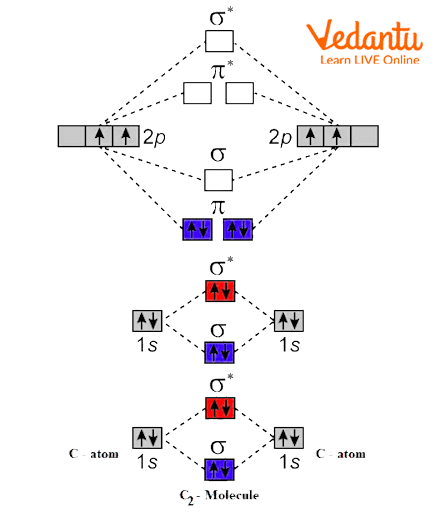2. Define a Coordinate Bond.

A coordinate bond (also known as a dative covalent bond) is a type of covalent connection (a shared pair of electrons) in which both electrons originate from the same atom. Two atoms sharing a pair of electrons make a covalent bond. Since both nuclei are attracted to the electron pair, the atoms are held together.

An electrophile forms coordinate connections with one or more nucleophiles arranged around it. To satisfy the octet rule, nucleophiles function as "ligands" by supplying two electrons per coordinate bond to the electrophile.

3. What are sigma bonds and pi bonds?

• Covalent bonds with overlapping atomic orbitals fall under the categories of sigma and pi bonds. Atomic orbitals overlapping results in covalent bonding.

• While pi bonds are created by the lateral overlapping of two atomic orbitals, sigma bonds are created by the head-to-head overlapping of atomic orbitals.

• The manner in which the overlapping of atomic orbitals occurs affects a number of bond properties, including bond length, bond angle, and bond enthalpy. Sigma and pi bonds are the two main forms of covalent bonds that are produced as a result of this overlap.

Comment